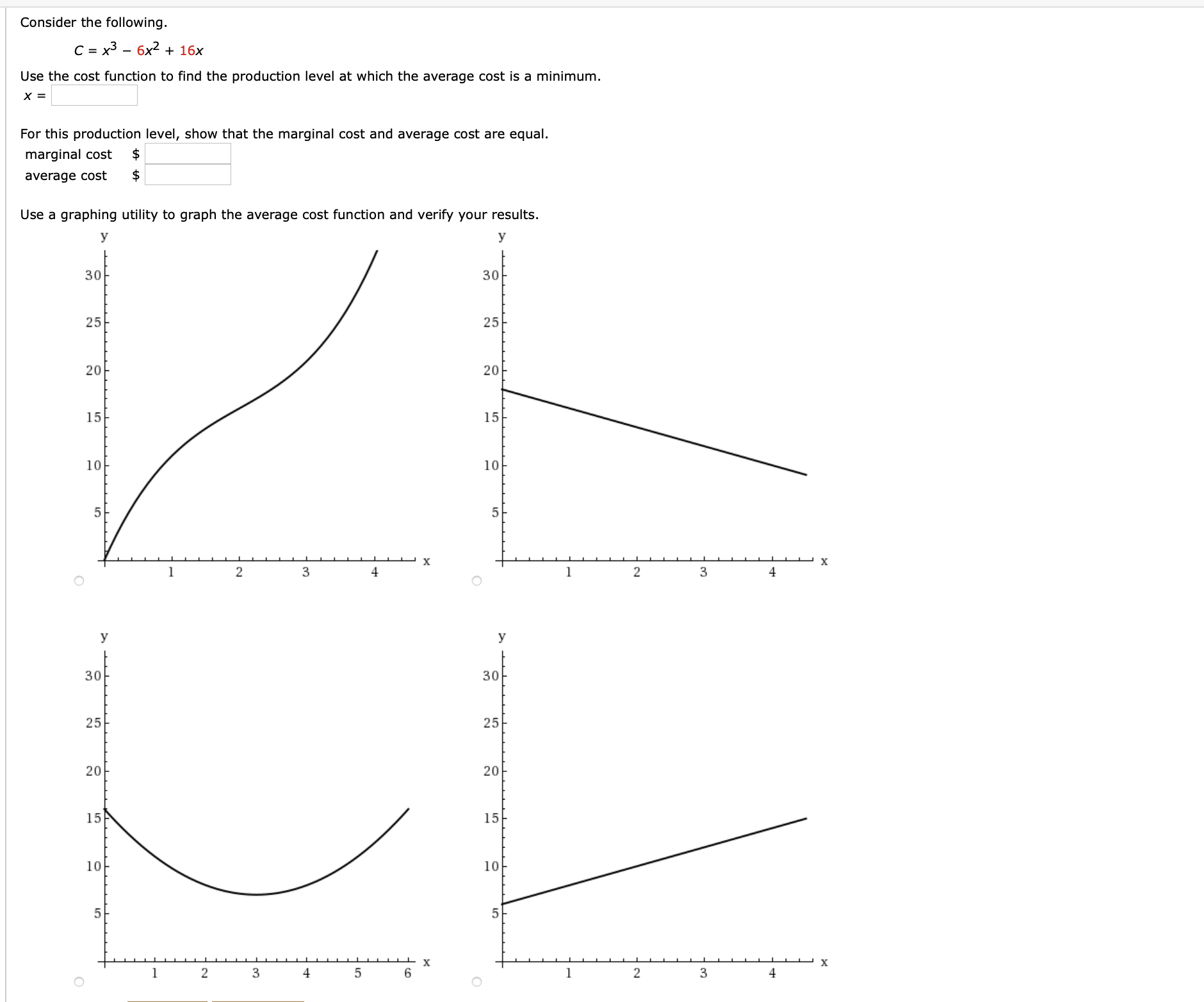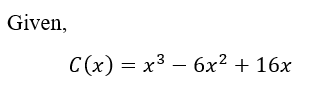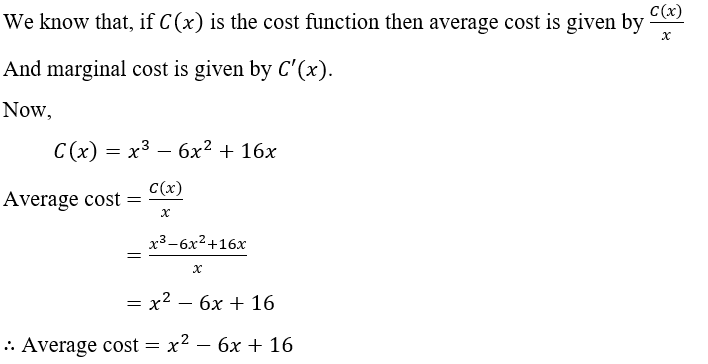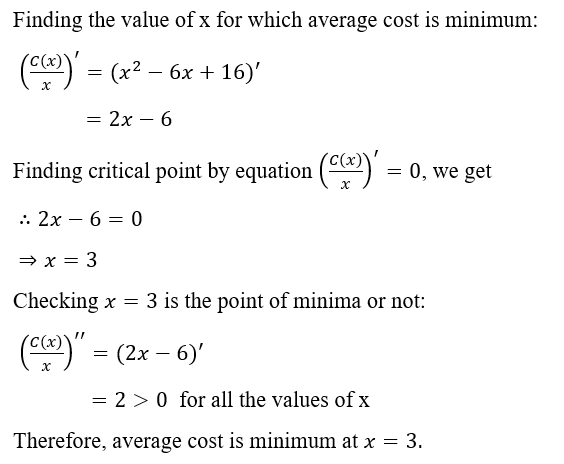# Consider the following.C = x3 – 6x2 + 16xUse the cost function to find the production level at which the average cost is a minimum.For this production level, show that the marginal cost and average cost are equal.marginal costaverage costUse a graphing utility to graph the average cost function and verify your results.У30302525-2020아1515101043y3030252520151510105хх2343420

Question
1 views

How do you solve the question in the attached image?help_outlineImage TranscriptioncloseConsider the following. C = x3 – 6x2 + 16x Use the cost function to find the production level at which the average cost is a minimum. For this production level, show that the marginal cost and average cost are equal. marginal cost average cost Use a graphing utility to graph the average cost function and verify your results. У 30 30 25 25- 20 20아 15 15 10 10 4 3 y 30 30 25 25 20 15 15 10 10 5 х х 2 3 4 3 4 20 fullscreen
check_circle

Step 1Step 2Step 3...

### Want to see the full answer?

See Solution

#### Want to see this answer and more?

Solutions are written by subject experts who are available 24/7. Questions are typically answered within 1 hour.*

See Solution
*Response times may vary by subject and question.
Tagged in